#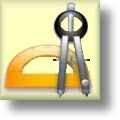Geometry Worksheets

## Parallel and Perpendicular Lines Worksheets

Here is a graphic preview for all of the Parallel and Perpendicular Lines Worksheets. You can select different variables to customize these Parallel and Perpendicular Lines Worksheets for your needs. The Parallel and Perpendicular Lines Worksheets are randomly created and will never repeat so you have an endless supply of quality Parallel and Perpendicular Lines Worksheets to use in the classroom or at home. We have identifying parallel lines, identifying perpendicular lines, identifying intersecting lines, identifying parallel, perpendicular, and intersecting lines, identifying parallel, perpendicular, and intersecting lines from a graph, Given the slope of two lines identify if the lines are parallel, perpendicular or neither, Find the slope for any line parallel and the slope of any line perpendicular to the given line, Find the equation of a line passing through a given point and parallel to the given equation, Find the equation of a line passing through a given point and perpendicular to the given equation, and determine if the given equations for a pair of lines are parallel, perpendicular or intersecting for your use. Our Parallel and Perpendicular Lines Worksheets are free to download, easy to use, and very flexible.

Click here for a Detailed Description of all the Parallel and Perpendicular Lines Worksheets.

## Quick Link for All Parallel and Perpendicular Lines Worksheets

Click the image to be taken to that Parallel and Perpendicular Lines Worksheet.

##### Identifying ParallelLines Worksheets##### Identifying PerpendicularLines Worksheets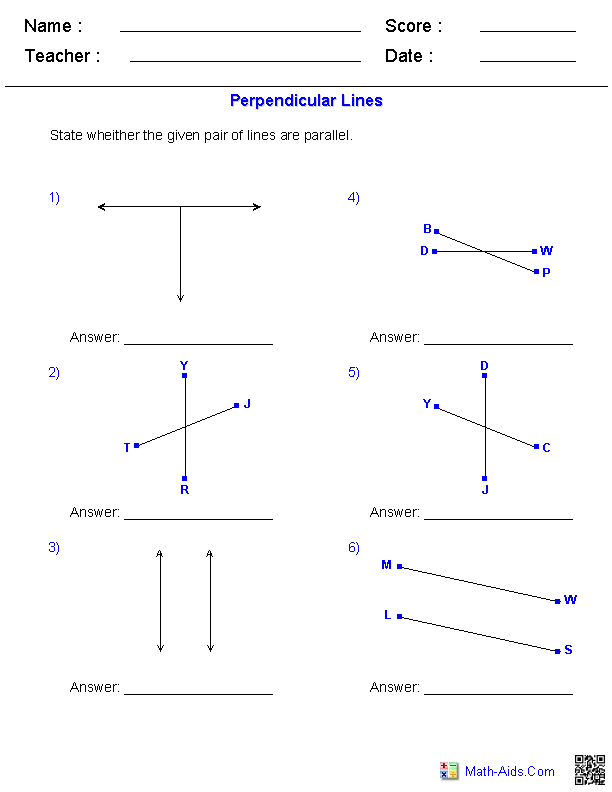##### Identifying Parallel,Perpendicular, & IntersectingLines Worksheets##### Identifying Parallel,Perpendicular, & IntersectingLines from a Graph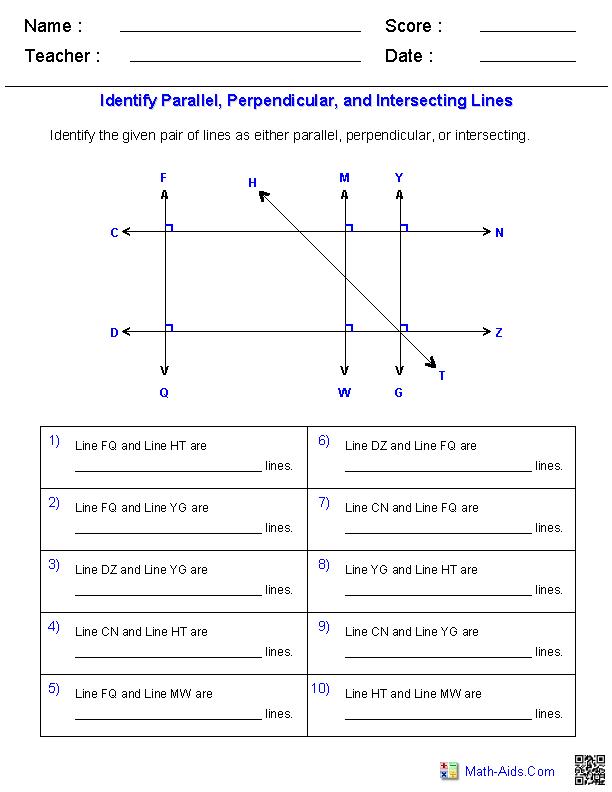##### Given Slopes IdentifyParallel, Perpendicular,or Neither Worksheets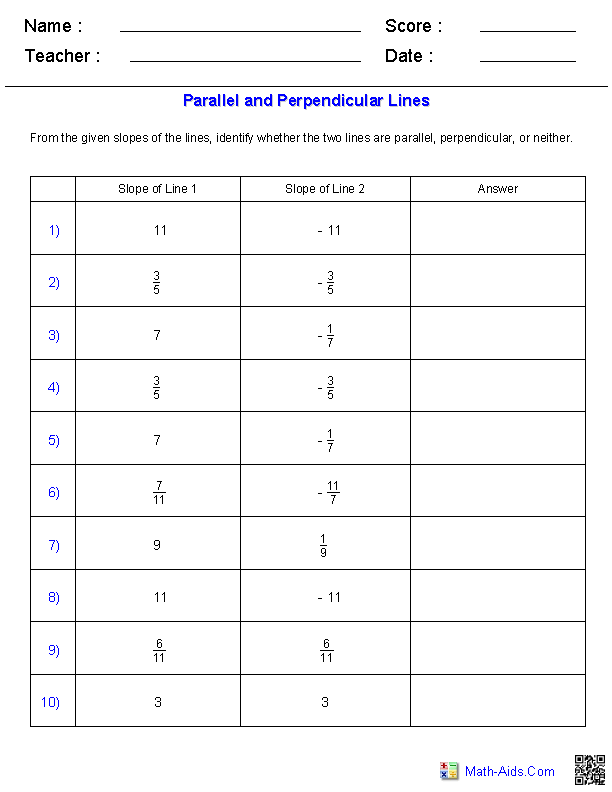##### Given Slope of a LineFind Slopes for Paralleland Perpendicular Lines##### Find the Equation of a ParallelLine Passing Through aGiven Equation and PointWorksheets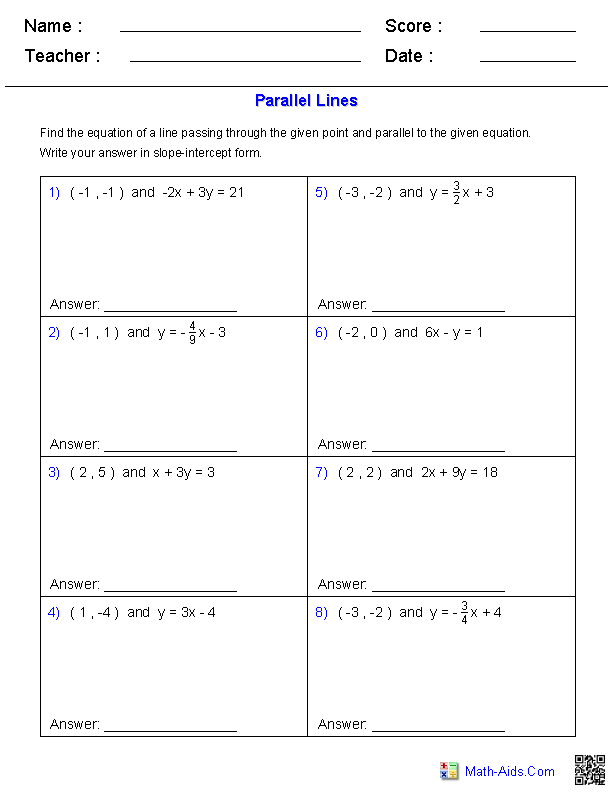##### Find the Equation of aPerpendicular Line PassingThrough a Given Equationand Point Worksheets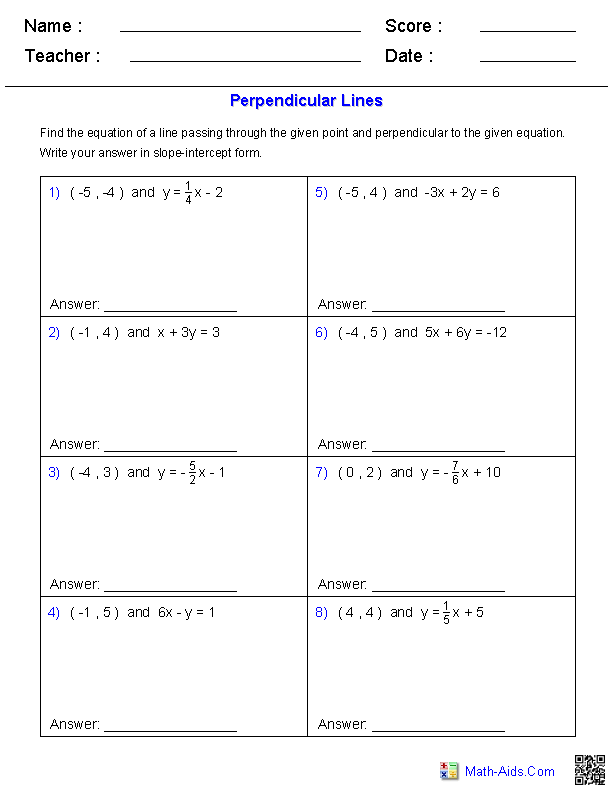##### Given a Pair of LinesDetermine if the Lines areParallel, Perpendicular,or Intersecting Worksheets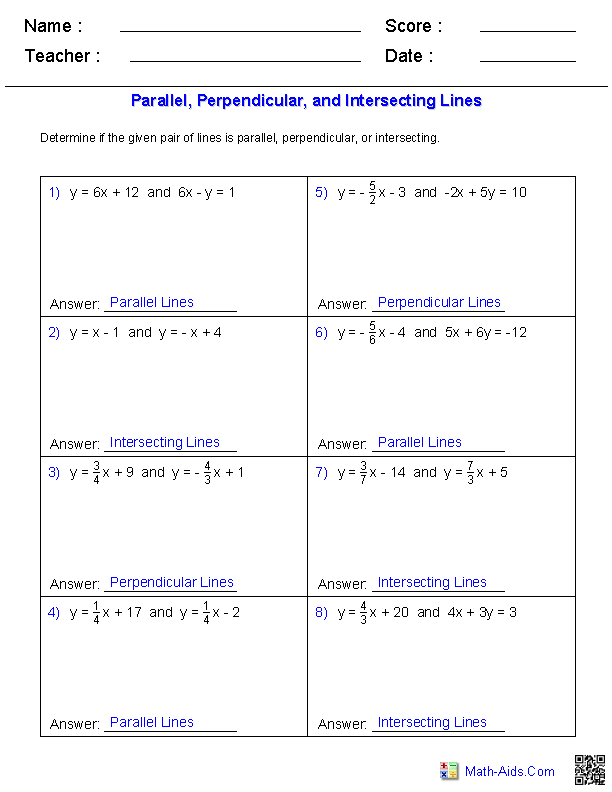Recommended Videos

## Detailed Description for All Parallel and Perpendicular Lines Worksheets

Identifying Parallel Lines Worksheets
These Parallel and Perpendicular Lines Worksheets are great for practicing identifying parallel lines from pictures. These worksheets will produce 6 problems per page.

Identifying Perpendicular Lines Worksheets
These Parallel and Perpendicular Lines Worksheets are great for practicing identifying perpendicular lines from pictures. These worksheets will produce 6 problems per page.

Identifying Parallel, Perpendicular, and Intersecting Lines Worksheets
These Parallel and Perpendicular Lines Worksheets are great for practicing identifying parallel, perpendicular, and intersecting lines from pictures. These worksheets will produce 6 problems per page.

Identifying Parallel, Perpendicular, and Intersecting Lines from a Graph
These Parallel and Perpendicular Lines Worksheets will show a graph of a series of parallel, perpendicular, and intersecting lines and ask a series of questions about the graph. These worksheets will produce 10 problems per page.

Given Slopes of Two Lines Determine if the Lines are Parallel, Perpendicular, or Neither
These Parallel and Perpendicular Lines Worksheets will give the slopes of two lines and ask the student if the lines are parallel, perpendicular, or neither. These worksheets will produce 10 problems per page.

Given Slope of a Line Find Slopes for Parallel and Perpendicular Lines Worksheets
These Parallel and Perpendicular Lines Worksheets will give the slope of a line and ask the student to determine the slope for any line that is parallel and the slope that is perpendicular to the given line. These worksheets will produce 10 problems per page.

Find the Equation of a Parallel Line Passing Through a Given Equation and Point
These Parallel and Perpendicular Lines Worksheets will ask the student to find the equation of a parallel line passing through a given equation and point. These worksheets will produce 6 problems per page.

Find the Equation of a Perpendicular Line Passing Through a Given Equation and Point
These Parallel and Perpendicular Lines Worksheets will ask the student to find the equation of a perpendicular line passing through a given equation and point. These worksheets will produce 6 problems per page.

Given a Pair of Lines Determine if the Lines are Parallel, Perpendicular, or Intersecting
These Parallel and Perpendicular Lines Worksheets will give the student a pair of equations for lines and ask them to determine if the lines are parallel, perpendicular, or intersecting. These worksheets will produce 6 problems per page.